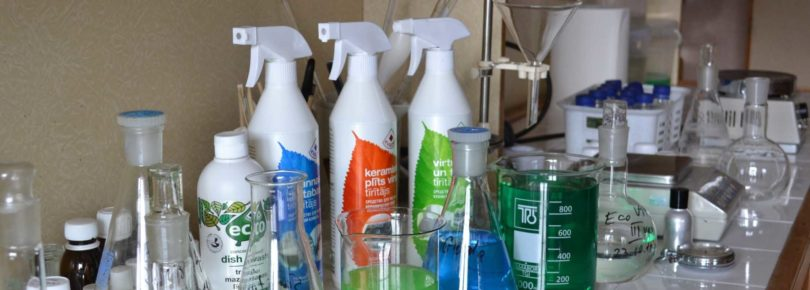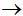Engineering Entrance Sample Papers

COMEDK UGET Chemistry Model Question PapersCOMEDK Undergraduate Entrance Test Chemistry model practice question paper consist 60 multiple choice questions with answers based on COMEDK syllabus and exam pattern.

Ques. 0.01 M solution of each of urea, salt and Na2SO4 is taken. The ratio of depression in freezing point will be
(a) 1:1:1
(b) 1:2:1
(c) 1:2:3
(d) 2:2:3
Ans. (c)

Ques. Molecular weight of a compound of an element is 125. If it reacts with three atoms of chlorine, then its equivalent weight will be approximately
(a) 124
(b) 41
(c) 60
(d) 50
Ans. (b)

Ques. What is molecular formula of 1.35 gm of phosphorus vapour of volume 500 ml at 300°C and 750 mm of Hg pressure
(a) P8
(b) P6
(c) P3
(d) P4
Ans. (d)

Ques. The main product of the nitration of propane is
(a) Nitropropane
(b) 2-Nitropropane
(c) Ethyl nitrite
(d) Nitroethane
Ans. (b)

Ques. Which of the following statements is correct for CsBr3
(a) It is a covalent compound
(b) It contains Cs3+ and Brions
(c) It contains Cs+ and Br3ions
(d) It contains Cs+, Brand lattice Br2 molecule
Ans. (c)

Ques. Crystal structure of Nacl is
(a) FCC
(b) BCC
(c) (a) and (b) both
(d) None of the above
Ans. (a)

Ques. According to position in the electrochemical series, which of the following metals cannot displace hydrogen form water and acids
(a) Al
(b) Hg
(c) Pb
(d) Ba
Ans. (b)

Ques. Increasing rate of dehydrohalogenation
(a) RF < RCl < RBr < RI
(b) RI < RBr < RCl < RF
(c) RCl < RF < RBr < RI
(d) RF < RI < RCl < RBr
Ans. (a)

Ques. Kp for the following reaction at 700 K is 1.3 x 10–3 atm-1. The Kc at same temperature for the reaction 2SO2 + O2 2SO3 will be
(a) 1.1 x 10–2
(b) 3.1 x 10–2
(c) 5.2 x 10–2
(d) 7.4 x 10–2
Ans. (d)

Ques. In compounds of type ECl3, where E = B, P, As or Bi, the angles Cl – E – Cl for different E are in the order
(a) B> P = As = Bi
(b) B > P > As > Bi
(c) B < P = As = Bi
(d) B < P < As < Bi
Ans. (b)

Ques. All of the following properties can be used for distinguishing alkyl cyanides and alkyl isocyanides, except
(a) Odour
(b) Reduction
(c) Hydrolysis
(d) Solubility in ether
Ans. (d)

Ques. Which of the following undergoes reaction with pheno, but not by aniline
(a) CH3COCl
(b) Br2 + H2O
(c) CH3Cl + AlCl3
(d) CH3I
Ans. (c)

Ques. Numbers of electrons in –CONH2 is
(a) 22
(b) 24
(c) 20
(d) 28
Ans. (b)

Ques. Which of the following pairs is correctly matched?
(a) CFCl3 – Freon – 111
(b) CF2Cl2 – Freon – 112
(c) C2F2Cl4 – Freon – 12
(d) C2F4Cl2 – Freon – 114
Ans. (d)

Ques. Which of the following molecular species has unpaired electron (s)
(a) N2
(b) F2
(c) O2
(d) O22–
Ans. (c)

Ques. Among the following species, identify the isostructural pairs NF3, NO3, BF3, H3O+, HN3
(a) [NF3, NO3] and [BF3, H3O+]
(b) [NF3, HN3] and [NO3, BF3]
(c) [NF3, H3O+] and [NO3, BF3]
(d) [NF3, H3O+] and [HN3, BF3]
Ans. (c)

Related: Maths questions for EAMCET

Ques. A sudden large jump between the values of second and third ionization energies of an element would be associated with the electronic configuration
(a) 1s2, 2s2 p6, 3s1
(b) 1s2, 2s2 p6, 3s2p1
(c) 1s2, 2s2 p6, 3s2p2
(d) 1s2, 2s2 p6, 3s2
Ans. (d)

Ques. Which of the following is obtained on adding nitrous acid to 2-nitropropane and shaking it with sodium hydroxide and ether
(a) Red aqueous solution
(b) Blue ethereal solution
(c) Colourless solution
(d) Milkiness
Ans. (b)

Ques. Benzenediazonium salt is converted to which compound in Balz-Schiemann reaction
(a) C6H5Br
(b) C6H5I
(c) C6H5Cl
(d) C6H5F
Ans. (d)

Ques. Volume of a gas NTP is 1.12 x 10–7 c.c. The number of molecules in it will be
(a) 3.1 x 1020
(b) 3.01 x 1012
(c) 30.1 x 1023
(d) 3.01 x 1024
Ans. (b)

Ques. pH will undergo maximum change on mixing which of the following solution in 10 ml 0.1 M KOH
(a) 10 ml 2.0 M HNO3
(b) 20 ml distilled water
(c) 10 ml 1.0 M HNO3
(d) 20 ml 0.5 M HNO3
Ans. (a)

Ques. Which of the following compounds is the product of the reaction of phenol and diazomethane?
(a) o-Cresol
(b) Methyl phenyl ether
(c) Phenyldiazomethane
(d) Phenetole
Ans. (b)

Ques. Concentrations of Pb+2 and Cu+ ions in a solution are 0.001 M and 0.01 M, respectively. Which of the following will be precipitated first on adding Clto it, if the Ksp values of PbCl2 and CuCl are 10–6 and 10–7, respectively
(a) CuCl2
(b) CuCl
(c) PbCl2
(d) Difficult to say
Ans. (b)

Ques. In a reaction 2A + BA2B, the reactant A will disappear at
(a) Half the rate that B will decrease
(b) The same rate that B will decrease
(c) Twice the rate that B will decrease
(d) The same rate that A2B will form
Ans. (c)

Ques. Benzaldehyde is reacted with which of the following compound in Knoevenagel reaction
(a) Acetic anhydride
(b) Malonic ester
(c) Acetaldehyde
(d) Acetone
Ans. (b)

Ques. The normal vapour density of PCl5 is 104.25. Its vapour density at 250°C when it is 80% dissociated is
(a) 52
(b) 57.9
(c) 86
(d) 120
Ans. (b)

Ques. If Ksp for HgSO4 is 6.4 x 10–5 then solubility of the salt is
(a) 8 x 10–3
(b) 8 x 10–6
(c) 6.4 x 10–5
(d) 6.4 x 10–3
Ans. (a)

Ques. Which of the following products is prepared from Benzylidyne chloride on hydrolysis by iron catalyst and lime water?
(a) Calcium benzoate
(b) Benzoic acid
(c) Benzyl alcohol
(d) Benzaldehyde
Ans. (b)

Ques. Which orbital has two angular nodal planes?
(a) s
(b) p
(c) d
(d) f
Ans. (c)

Ques. Which of the following will undergo reaction to form a tertiary amine?
(a) MeOH + MeNH2
(b) Me2NH + MeI
(c) Me3C – Cl + NH3
(d) (CH3)3C – MgCl + NH2Cl
Ans. (b)

Related: COMEDK Math Sample Paper

Ques: Methyl isocyanide on hydrolysis gives
(a) CH3NH2
(b) HCOOH
(c) CH3COOH
(d) Both (a) and (b)
Ans: (d)

Ques. An oxide of metal (M) has 40% by mass of oxygen. Metal M has relative atomic mass of 24. The empirical formula of the oxide is
(a) M2O
(b) M2O3
(c) MO
(d) M3O4
Ans. (c)

Ques. The reagent appropriate for conversion of a primary amine directly to an alkyl chloride is associated with the following name
(a) Hinsberg
(b) Tilden
(c) Grignard
(d) Hofmann
Ans. (b)

Ques. For the following homogeneous  gas reaction 4NH3 + 5O2 ⇌ 4NO + 6H2O, the equilibrium constant Kc has the dimension of
(a) Conc+10
(b) Conc+1
(c) Conc–1
(d) It is dimensionless
Ans. (b)

Ques. Which of the following classes of compounds is formed on alkaline hydrolysis of methyl isocyanate?
(a) 3° Amine
(b) 2° Amine
(c) 1° Amine
(d) Ammonium salt
Ans. (c)

Ques. Which pair of elements has same chemical properties?
(a) 13, 22
(b) 3, 11
(c) 4, 24
(d) 2, 4
Ans. (b)

Ques. Standard reduction potential of metallic electrodes, A, B, C and D, are +0.14V, +0.34V, –0.74V and –0.4V, respectively. Decreasing order of their reducing capacity will be
(a) C > D > A > B
(b) A > B > C > D
(c) A > C > D > B
(d) C > B > A > D
Ans. (a)

Ques. In a secondary amine
(a) One – NH2 group is attached on a secondary carbon atom
(b) Two – NH2 groups are attached on the same carbon atom
(c) One – NH – group is attached to two alkyl groups
(d) Two – NH2 groups are present
Ans. (c)

Ques. For hydrogen gas Cp – Cv = a, and for oxygen gas Cp – Cv = b, so the relation between a and b is
(a) 1 = 16b
(b) 16a = b
(c) a = 4b
(d) a = b
Ans. (d)

Ques. Which of the following elements will have the lowest first ionization energy ?
(a) Mg
(b) Rb
(c) Li
(d) Ca
Ans. (b)

Ques. Which of the following is formed on fusing solid caustic potash in nitrobenzene?
(a) An SE product
(b) A mixture of two SE products
(c) Phenol
(d) A mixture of two SN products
Ans. (d)

Related: COMEDK UGET Guide

Ques. Which of the following products is obtained on exposing a mixture of benzene and chlorine to ultraviolet light?
(a) Benzene hexachloride
(b) Hexachlorobenzene
(c) 1,3,5-Trichlorobenzene
(d) Benzotrichloride
Ans. (a)

Ques. Which of the following attacks glass
(a) HCl
(b) HF
(c) HI
(d) HBr
Ans. (b)

Ques. O.N. of P in KH2PO2 is
(a) +1
(b) +3
(c) +5
(d) –4
Ans. (a)

Ques. A sample of H2SO4 (density 1.8 g/ml) is 90% by weight. What is the volume of the acid that has to be used to make 1 litre of 0.2 M H2SO4
(a) 16 ml
(b) 10 ml
(c) 12 ml
(d) 18 ml
Ans. (c)

Ques. Which of the following is formed on complete hydrolysis of acetaldehyde cyanohydrins
(a) Citric acid
(b) Tartaric acid
(c) Malic acid
(d) Lactic acid
Ans. (d)

Ques. Which of the following names is incorrect for (CH2)6N4
(a) Hexamine
(b) Aminoform
(c) Urotropine
(d) Hexametheylenedraiamine
Ans. (d)

Ques. In what regard, an organic catalyst is different from an inorganic catalyst
(a) It acts at low temperature
(b) It is proteinous in nature
(c) It acts by different mechanism
(d) It acts at high temperatures
Ans. (b)

Ques. Which of the following compounds gives ethyl with nitrogen pentoxide to give acetyl nitrate?
(a) Acetyl chloride
(b) Acetic anhydride
(c) Acetamide
(d) Ethyl acetate
Ans. (b)

Ques. A salt of sulphurous acid is called
(a) Sulphate
(b) Sulphurate
(c) Sulphite
(d) Sulphide
Ans. (c)

Ques. Which of the following ions form most stable complex compound
(a) Cu++
(b) Ni++
(c) Fe++
(d) Mn++
Ans. (a)

Ques. Nitrogen and the nitride ion have the
(a) Same size
(b) Same number of electrons
(c) Same electrons configuration
(d) Same number of protons
Ans. (d)

Ques. Electron having : m = +1 and s = – ½ cannot belong to the following orbital
(a) p
(b) d
(c) f
(d) s
Ans. (d)

Ques. Which one of the following is more acidic
(a) Butane
(b) 1-butene
(c) 1-butyne
(d) 2-butyne
Ans. (b)

Ques. How many ml of 0.1 M oxalic acid solution is required to reduce 0.01 mole KMnO4 to MnO2 is
(a) 250
(b) 150
(c) 100
(d) 500
Ans. (b)

Ques. Which one of the following is smallest in size ?
(a) N3–
(b) O2–
(c) F
(d) Na+
Ans. (d)

Ques. A reducing agent is
(a) Electrons acceptor
(b) Electrons donor
(c) Protons donor
(d) None of these
Ans. (b)

Ques. The following equilibrium exists in aqueous solution CH3COOH ⇌ CH3COO + H+. If dilute HCl is added without a change in temperature, then the
(a) Concentration of CH3COO will increase
(b) Concentration of CH3COOwill decrease
(c) Equilibrium constant will increase
(d) Equilibrium constant will decrease
Ans. (b)

Ques. The property by virtue of which a compound can turn the plane polarised light is known as
(a) Photolysis
(b) Phosphorescence
(c) Optical activity
(d) Polarization
Ans. (c)

Ques. When ethyl alcohol is heated with red phosphorus and HI, then which of the following is formed
(a) C2H6
(b) CH4
(c) C3H8
(d) C2H4
Ans. (a)

Ques. Purification of a colloid can be done by which of the following
(a) Peptisation
(b) Dialysis
(c) Coagulation
(d) Condensation
Ans. (b)